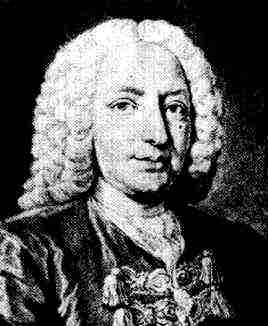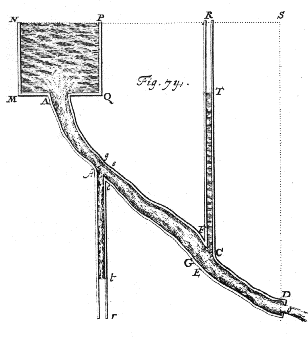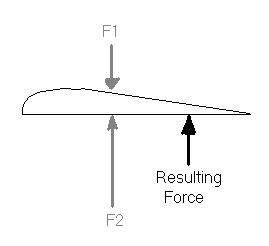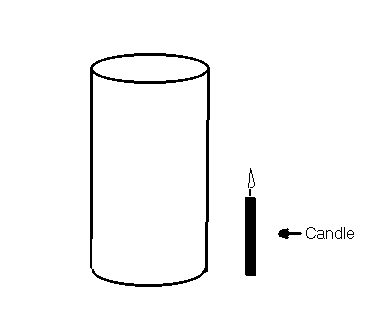Daniel BernoulliDaniel Bernoulli was born on January 29, 1700.

Growing up, he wanted to study calculus. His father however had other plans. He wanted him to be a merchant, as he thought that there was no money to be made in mathematics. In the end they reached an agreement and he became a doctor. He began doing research on ways of measuring blood pressure. He would insert a glass pipe into an artery and use the height to determine the pressure in the artery. This is a diagram of the experiment.From experiments like this we get the following equation.

P1 + 1/2rv12 + rgh1 = P2 + 1/2rv22 + rgh2

Since for a wing the boundary layers for the top and bottom of the wing are at virtually the same height and the air is assumed to have the same density everywhere we can rewrite the above equation as follows:

P1 + 1/2rv12 = P2 + 1/2rv22

We can use this equation to discover how a lift force can be generated over a wing when the angle of attack is small (~5°).  Zero attack angle means lift force is zero.

Paper Demo

We can demonstrate how this works by blowing over a piece of paper. When we do this the paper rises.

We can see here that the air is traveling faster on top (1) than on the bottom (2) and by the equation we can see    P1 < P2

P = F/A or F = PA

So then from this F1 < F2 and there will be a net force up.For this concept to work the air needs to "adhere" to the surface of the wing. There needs to be a boundary layer of air flowing around the wing. This can be counter intuitive for a grade 12 student, so to show that this is what actually happens. The following demonstration was used.

Candle Demo

Set up the following apparatus.Blow out the candle by blowing on the cylinder. The candle will be blown out. This reinforces the idea the air "adheres" to the wing.

Main Demo

Using the apparatus shown in the construction section we can show how there is a lift force generated when the attack angle is small.

Set the wing to 90° on the protractor on the cart and 90° on the vertical protractor.

Turn on the wind generator and let the wing on the cart move freely. It will eventually start to move because of the wind. This is helpful to the students to get a direct visual of what is going on. Next attach a spring and hold the cart (spring unstretched). Turn the wind generator on and pull on the spring to make sure the cart always stays in the same place. The length that the spring stretches can be used to give an idea of relative forces as F = -kx. Note the length of the spring.